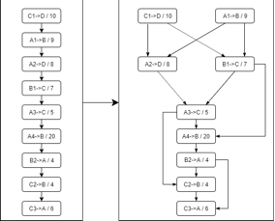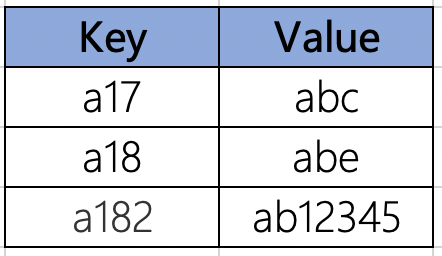# Highlight of PlatON 0.13.0 – Enhancing Performance Using Parallel Transactions Processing and Parallel Merkle Root Calculation

Like most of the mainstream public blockchains, in versions prior to PlatON 0.13.0, transactions were executed sequentially, which is safer because previous transactions can affect the execution of the current transaction. As the contents of parent nodes in the trees are the hashes of the children nodes, concurrency in merkle trees is hard to achieved. The current standard for merkle tree updates is a naive locking of the entire tree, and so is PlatON.

These two serial methods limit the system throughput and increases transaction acceptance latency, and they are even unable to exploit the modern multi-core resources efficiently

However, some transactions are disjoint or independent, meaning that they do not write on the same state variables during their execution. These independent transactions can be executed parallelly without any conflict with other transactions. The state variables rewritten by these transactions may also be on different branches of the merkle tree. Before calculating the merge nodes, these branches can be calculated parallelly.

The underlying chain of PlatON 0.13.0 uses the DAG (directed acyclic graph) technology to execute transactions and calculate merkle root parallelly. The test results show that the overall performance is enhanced by 30%.

Parallel Transactions Processing

A directed acyclic graph (DAG) is accessed that is constructed based on inter-dependencies among the transactions. Whether the two transactions are dependent depends on whether the respective participants have intersection. The transactions for the new block are divided into a set of two or more independent tasks that can be executed in parallel based on the DAG. The independent tasks that can be managed independently are executed. All transactions of which the in-degree are 0 can be executed in parallel. Below is a DAG of transactions:Parallel Merkle Root Calculation

When the merkle tree is updated incrementally, the branches that need to be recalculated are generally disjoint or independent. A directed acyclic graph (DAG) is accessed that is constructed based on inter-dependencies among the nodes of these independent branches. Based on the DAG, the nodes that need to be recalculated are divided into a set of two or more independent tasks that can be equally distributed to n-threads where they calculate the hash of the adjacent pairs of nodes and return the result. This process is repeated until the merkle root is found.

Assume we insert the following state variables into the merkle tree:Then we can get an MPT tree shown as follows, which can generate the related DAG where all nodes of which the in-degree are 0 can calculated in parallel.

Then we can get an MPT tree shown as follows, which can generate the related DAG where all nodes of which the in-degree are 0 can calculated in parallel.

It is obvious that the performance of parallel version is higher than the one of serial version. PlatON will keep improving the performance indexes on top of ensuring the security and stability to provide users better user experience and services.

1 Like